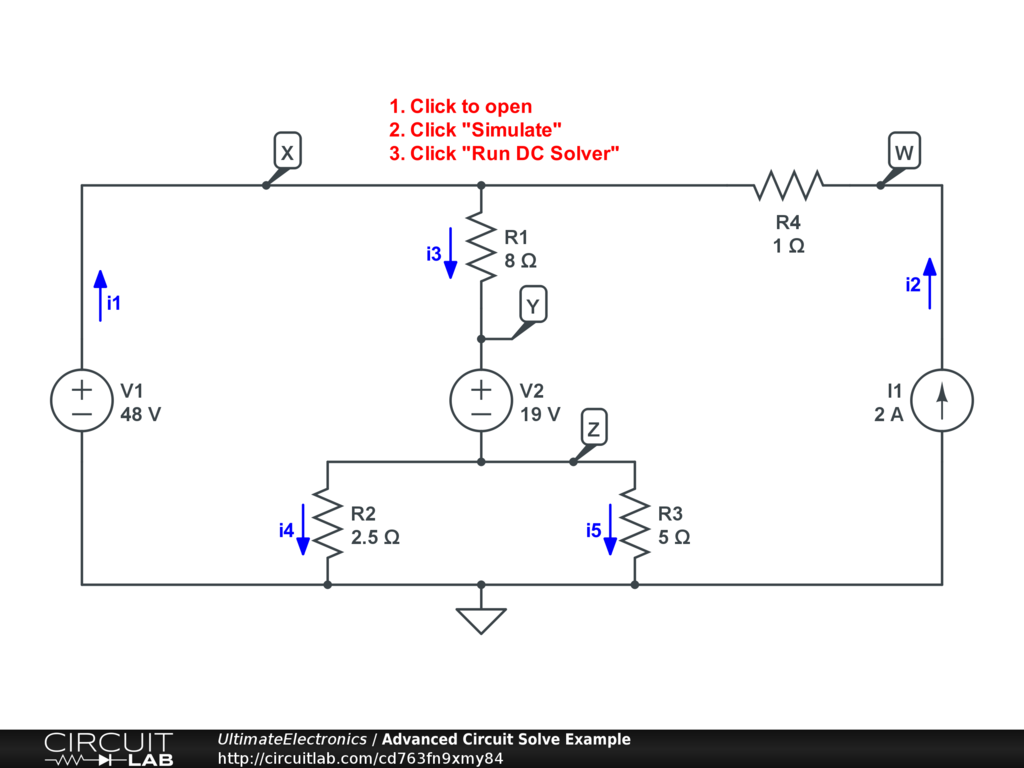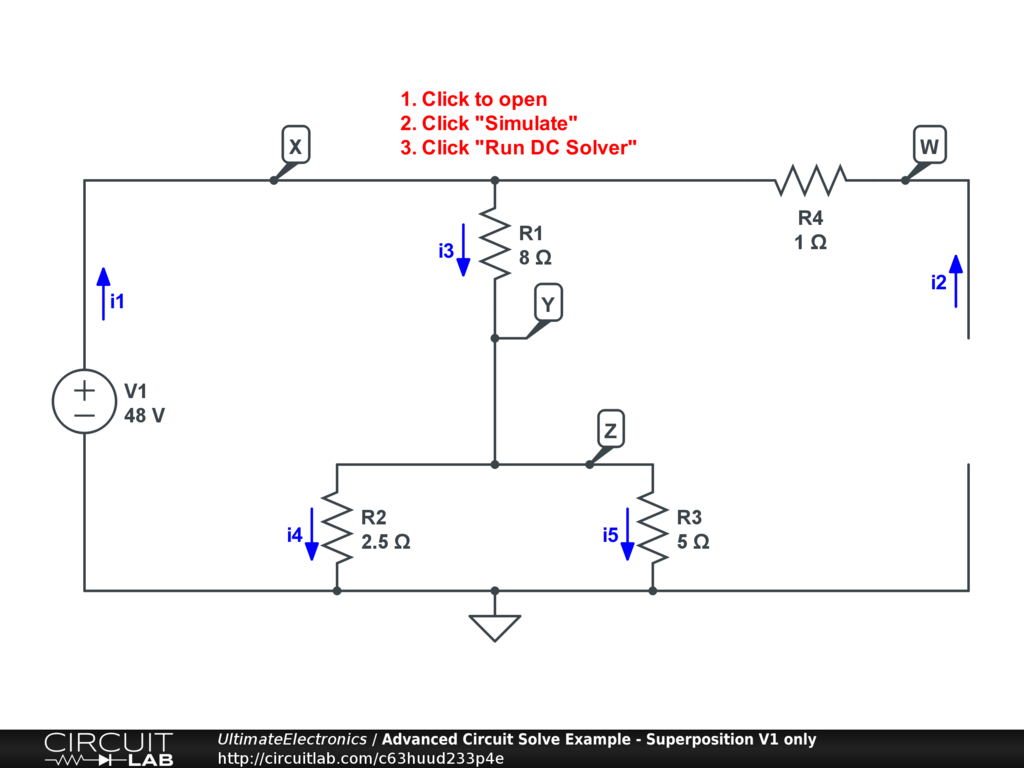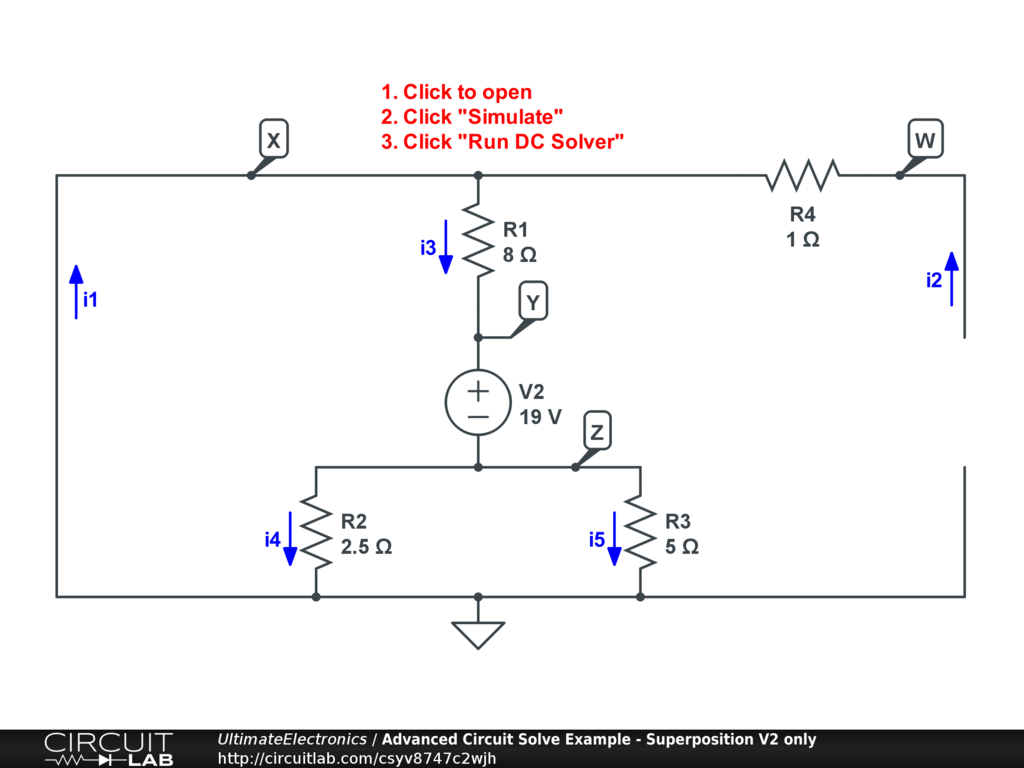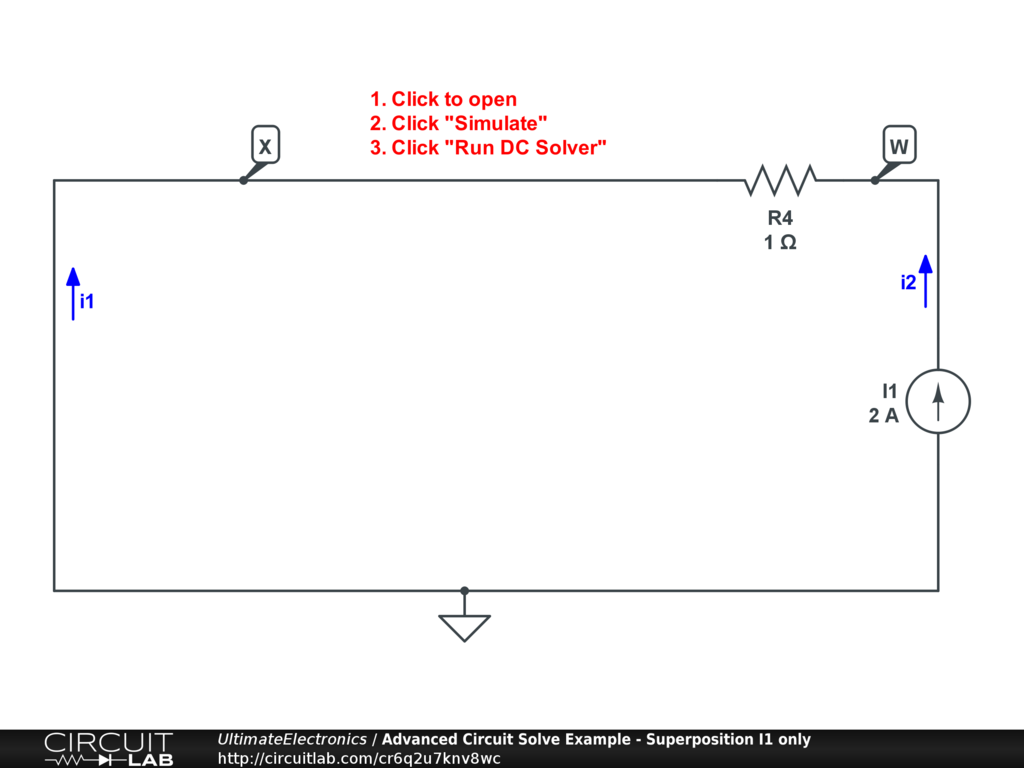Superposition

It should be clear that for any one-variable equation $y = m x$, then:

\begin{align} y_1 & = m x_1 \\ y_2 & = m x_2 \\ (y_1 + y_2) & = m (x_1 + x_2) \\ \end{align}

which holds true for any nonzero slope $m \ne 0$. In fact, a more general rule is true:

$$(a y_1 + b y_2) = m (a x_1 + b y_2)$$

This linear combination rule is what defines a linear system. As previously discussed, a consequence of linear systems and basic linear algebra is that a system of the a system of the form:

$$\mathbf{A} \mathbf{x} = \mathbf{y}$$

where $\mathbf{A}$ is any square invertible matrix, then the same result holds. If the solution to for some right-hand-side vector $\mathbf{y_1}$ is the vector $\mathbf{x_1}$, and so on, then:

$$\mathbf{A} (c_1 \mathbf{x_1} + c_2 \mathbf{x_2}) = (c_1 \mathbf{y_1} + c_2 \mathbf{y_2})$$

A Matrix Example

Consider the following system of two equations and two unknowns:

\begin{align} 2 m + n & = 5 \\ m - n & = 1 \end{align}

This can be equivalently represented as a matrix equation:

\begin{align} \mathbf{A} \mathbf{x} & = \mathbf{y} \\ \begin{bmatrix} 2 & 1 \\ 1 & -1 \end{bmatrix} \begin{bmatrix} m \\ n \end{bmatrix} & = \begin{bmatrix} 5 \\ 1 \end{bmatrix} \end{align}

This system has the solution $m=2, \ n=1$, or:

$$\mathbf{x}=\begin{bmatrix}m\\n\end{bmatrix}=\begin{bmatrix}2\\1\end{bmatrix}$$

(For review on how we got there, see Systems of Equations.)

We could have solved this system using superposition by breaking up the right-hand-side $\mathbf{y}=\begin{bmatrix}5 \\ 1\end{bmatrix}$ into parts for each nonzero source term: $\mathbf{y_1}=\begin{bmatrix}5 \\ 0\end{bmatrix}$ and $\mathbf{y_2}=\begin{bmatrix}0 \\ 1\end{bmatrix}$, so $\mathbf{y} = \mathbf{y_1} + \mathbf{y_2}$. In this case, we've left it so each one has just one non-zero cell.

Here's how we'd solve $\mathbf{A} \mathbf{x} = \mathbf{y}$ using our split $\mathbf{y} = \mathbf{y_1} + \mathbf{y_2}$:

1. Solve $\mathbf{A} \mathbf{x_1} = \mathbf{y_1}$ for $\mathbf{x_1}$.
2. Solve $\mathbf{A} \mathbf{x_2} = \mathbf{y_2}$ for $\mathbf{x_2}$.
3. Combine $\mathbf{x} = \mathbf{x_1} + \mathbf{x_2}$.

Let's work through the three steps.

For $\mathbf{x_1}$:

\begin{align} \mathbf{A} \mathbf{x_1} & = \mathbf{y_1} \\ \begin{bmatrix} 2 & 1 \\ 1 & -1 \end{bmatrix} \begin{bmatrix} m_1 \\ n_1 \end{bmatrix} & = \begin{bmatrix} 5 \\ 0 \end{bmatrix} \end{align}

This is easy to solve because the 2nd row tells us $m_1=n_1$, after which the first row tells us $3 m_1 = 5$, so $m_1 = \frac {5} {3}$. This component of the superposition has the solution:

$$\mathbf{x_1}=\begin{bmatrix}\frac {5} {3}\\ \frac{5} {3}\end{bmatrix}$$

For $\mathbf{x_2}$:

\begin{align} \mathbf{A} \mathbf{x_2} & = \mathbf{y_2} \\ \begin{bmatrix} 2 & 1 \\ 1 & -1 \end{bmatrix} \begin{bmatrix} m_2 \\ n_2 \end{bmatrix} & = \begin{bmatrix} 0 \\ 1 \end{bmatrix} \end{align}

In this case, the first equation tells us $n_2 = -2 m_2$, from which we can see that the second equation says $3 m_2 = 1$, so $m_2 = \frac {1} {3}$, and then $n_2 = - \frac {2} {3}$. This component of the superposition has the solution:

$$\mathbf{x_2}=\begin{bmatrix}\frac {1} {3}\\ - \frac{2} {3}\end{bmatrix}$$

Combine the Parts:

\begin{align} \mathbf{x} & = \mathbf{x_1} + \mathbf{x_2} \\ & = \begin{bmatrix}\frac {5} {3}\\ \frac{5} {3}\end{bmatrix} + \begin{bmatrix}\frac {1} {3}\\ - \frac{2} {3}\end{bmatrix} \\ & = \begin{bmatrix} 2 \\ 1 \end{bmatrix} \end{align}

As expected, the sum matches our directly-solved answer above.

Notice that for both sub-problems, solving the system of equations was made much easier because equations with zero right-hand-side quickly told us that two variables are simply proportional to each other, allowing us to reduce the solution space rapidly.

This is how superposition works on a mathematical level. It's a divide-and-conquer problem solving technique that lets us split a linear system problem into simpler subproblems, from which we can easily combine subsolutions to find our full solution later.

Sources to Equations

As you may have noticed in Solving Circuit Systems, there were only two components capable of producing a non-zero right-hand-side value in their equations: voltage sources and current sources. Resistors, for example, always generate a component constitutent equation with zero right-hand-side value, as they related a branch current and a voltage difference with intercept at the origin. Additionally, equations from Kirchhoff's Current Law always sum to zero.

As a result, we can apply superposition to all independent sources, considering the circuit with only one on at a time (setting the rest to 0), and later combine all the node voltages and branch currents to find the final operating point of the circuit.

By turning a source "off," we mean setting its value to zero. This has slightly different meaning for voltage and current sources:

• For a voltage source, "off" means 0 volts voltage difference ($v_A - v_B = 0$), which is a short circuit. We can replace the voltage source with a wire as though the two terminals are directly connected.
• For a current source, "off" means 0 amps flowing through ($i_X = 0$), which is an open circuit. We can delete the current source and leave the two terminals unconnected.

These may seem like opposite outcomes, but remember that both are the straightforward result of setting the right-hand side of their constitutent equations to zero.

Note that superposition must be treated specially in two cases:

1. Nonlinear elements. Nonlinear elements can have nonzero right-hand-side of their equations, in which case doing superposition only over the independent sources would not work. However, we can use superposition on the linearized model of a nonlinear circuit around a particular operating point, and this can be a useful technique.
2. Dependent sources. Later, we'll introduce sources which are not independent, but instead have some voltage or current which is proportional to some other branch current or voltage difference elsewhere in the circuit. Ideal dependent sources have zero right-hand-side as well, so we shouldn't think about turning these on and off. We'll address these later in the Superposition with Dependent Source section.

A Solved Superposition Example

In the Solving Circuit Systems section we looked at a circuit with 4 node voltages and 5 branch currents to solve for. We set up and solved the 9x9 system of equations considering all three sources simultaneously:Interactive Exercise Click to open and simulate the circuit above.

Now, however, we're going to solve the system by considering the system for each of the three independent sources (only one on at a time):

For voltage source V1:

We leave V1 on, but turn off V2 by replacing it by a wire (making $V_Y = V_Z$ be the same node), and turn I1 off by deleting it entirely (so its branch current $i_2 = 0$). Here's what this version of the circuit looks like:Interactive Exercise Click to open and simulate the circuit above.

This version of the circuit is very easy to solve by hand: $V_X=48$ by inspection, and $V_W = V_X$ because no current flow through R4. We have just one voltage source with three resistors, and a total equivalent resistance of

\begin{align} R_{\text{eq}} & = R1 + R2 // R3 \\ & = 8 + \frac {2.5 \cdot 5} {2.5 + 5} \\ & = \frac {29} {3} \end{align}

The total current from the voltage source is:

$$i_1 = i_3 = \frac {48} { \left( \frac{29} {3} \right) } = \frac {144} {29} \approx 4.966$$

From there, we can find the voltage drop in R1, so:

$$V_Y = V_Z = 48 - \frac {144} {29} \cdot 8 = \frac {240} {29} \approx 8.276$$

The total current $i_3$ splits between R2 and R3, and since one resistance is half the other, they will share the load two-thirds to one-third:

\begin{align} i_5 & = \frac{1} {3} i_3 = \frac {144} {87} \approx 1.655 \\ i_4 & = \frac{2} {3} i_3 = \frac {288} {87} \approx 3.310 \end{align}

That's it! The full solution vector for source V1 only is:

$$\begin{equation} \mathbf{x_{\text{V1}}} = \begin{bmatrix} i_1 \\ i_2 \\ i_3 \\ i_4 \\ i_5 \\ V_W \\ V_X \\ V_Y \\ V_Z \end{bmatrix} = \begin{bmatrix} \frac {144} {29} \\ 0 \\ \frac {144} {29} \\ \frac {288} {87} \\ \frac {144} {87} \\ 48 \\ 48 \\ \frac {240} {29} \\ \frac {240} {29} \end{bmatrix} \approx \begin{bmatrix} 4.966 \\ 0 \\ 4.966 \\ 3.310 \\ 1.655 \\ 48 \\ 48 \\ 8.276 \\ 8.276 \end{bmatrix} \end{equation}$$

The fractions aren't pretty, but the circuit becomes so simple that is easy to solve by hand without breaking out any real linear algebra.

You can quickly verify these calculations by clicking on the circuit above, then click "Simulate," and "Run DC Solver."

For voltage source V2:

In this case, we leave V2 on, but turn off V1 by replacing it with a wire (so X becomes a ground node), and turn off I1 by deleting it.Interactive Exercise Click to open and simulate the circuit above.

This version of the circuit is also fairly easy to reduce by hand. As X is now a ground node, $V_X = 0$, and since no current flows through resistor R4 as $i_2 = 0$, $V_W = 0$ also.

We now have a single voltage source V2 driving current around a single loop (in direction opposite to $i_3$) with a total resistance $R_{\text{eq}} = \frac {29} {3}$ just as earlier. (If this is too much of a leap, you can also solve the system quickly in full.) The total current will be:

$$i_1 = i_3 = - \frac {19} { \left( \frac{29} {3} \right) } = - \frac {57} {29} \approx -1.966$$

Again, the total current $i_3$ splits two-thirds to one-thirds between R2 and R3, so

\begin{align} i_5 & = \frac{1} {3} i_3 = - \frac {57} {87} \approx -0.655 \\ i_4 & = \frac{2} {3} i_3 = - \frac {114} {87} \approx -1.310 \end{align}

We can calculate the voltage at nodes Y and Z from Ohm's Law:

\begin{align} V_Y & = - 8 i_3 = -8 \cdot(- \frac {57} {29}) = \frac {456} {29} \approx 15.724 \\ V_Z & = V_Y - 19 = - \frac {95} {29} \approx -3.276 \end{align}

That's it! The full solution vector for source V2 only is:

$$\begin{equation} \mathbf{x_{\text{V2}}} = \begin{bmatrix} i_1 \\ i_2 \\ i_3 \\ i_4 \\ i_5 \\ V_W \\ V_X \\ V_Y \\ V_Z \end{bmatrix} = \begin{bmatrix} - \frac {57} {29} \\ 0 \\ - \frac {57} {29} \\ - \frac {114} {87} \\ - \frac {57} {87} \\ 0 \\ 0 \\ \frac {456} {29} \\ - \frac {95} {29} \end{bmatrix} \approx \begin{bmatrix} -1.966 \\ 0 \\ -1.966 \\ -1.310 \\ -0.655 \\ 0 \\ 0 \\ 15.724 \\ -3.276 \end{bmatrix} \end{equation}$$

Again, you can confirm these values numerically by clicking the circuit above, clicking "Simulate," and "Run DC Solver."

For current source I1:

We turn off V1 and V2 by setting their voltages to zero, or replacing them with a wire.Interactive Exercise Click to open and simulate the circuit above.

When we turn off V1 and V2, the circuit becomes very simple, as we can effectively remove resistors R1, R2, and R3 because no current will flow through them ($i_3=i_4=i_5=0$). The zero-resistance short circuit path due to V1 will always carry the full current between node X and ground (now that X is a ground!), rather than the finite-resistance path through the resistors.

This circuit is super easy to solve: $i_2 = 2$ and $V_W = 2$. The branch current $i_1$ is the same as (but in the opposite direction of) $i_2$, so $i_1 = -i_2 = -2$. The full solution vector for source I1 only is:

$$\begin{equation} \mathbf{x_{\text{I1}}} = \begin{bmatrix} i_1 \\ i_2 \\ i_3 \\ i_4 \\ i_5 \\ V_W \\ V_X \\ V_Y \\ V_Z \end{bmatrix} = \begin{bmatrix} -2 \\ 2 \\ 0 \\ 0 \\ 0 \\ 2 \\ 0 \\ 0 \\ 0 \end{bmatrix} \end{equation}$$

Combine the Parts:

Each of the three sub-problems was quite easy to solve -- much easier than the original problems. Now, we can use superposition to combine the three solution vectors into a single one:

\begin{align} \mathbf{x} & = \mathbf{x_{\text{V1}}} + \mathbf{x_{\text{V2}}} + \mathbf{x_{\text{I1}}} \\ \mathbf{x} & = \begin{bmatrix} \frac {144} {29} \\ 0 \\ \frac {144} {29} \\ \frac {288} {87} \\ \frac {144} {87} \\ 48 \\ 48 \\ \frac {240} {29} \\ \frac {240} {29} \end{bmatrix} + \begin{bmatrix} - \frac {57} {29} \\ 0 \\ - \frac {57} {29} \\ - \frac {114} {87} \\ - \frac {57} {87} \\ 0 \\ 0 \\ \frac {456} {29} \\ - \frac {95} {29} \end{bmatrix} + \begin{bmatrix} -2 \\ 2 \\ 0 \\ 0 \\ 0 \\ 2 \\ 0 \\ 0 \\ 0 \end{bmatrix} \\ \mathbf{x} & = \begin{bmatrix} 1 \\ 2 \\ 3 \\ 2 \\ 1 \\ 50 \\ 48 \\ 24 \\ 5 \end{bmatrix} = \begin{bmatrix} i_1 \\ i_2 \\ i_3 \\ i_4 \\ i_5 \\ V_W \\ V_X \\ V_Y \\ V_Z \end{bmatrix} \end{align}

This is exactly the same solution as we found when solving the circuit all at once in Solving Circuit Systems.

By using superposition, we were able to break a more complicated circuit down into three much easier ones. Their solutions were easily combined by addition.

Note that while we applied this technique to the entire solution vector of all node voltages and branch currents, the same concept applies for any individual value. For example, if we were only interested in the value of the node voltage $V_W$, we could apply superposition to quickly see the contribution of each source, without necessarily having to solve for all currents and voltages.

What's Next

In the next section, Open Circuit and Short Circuit, we'll talk about these two common terms and how they're applied to understanding circuits.